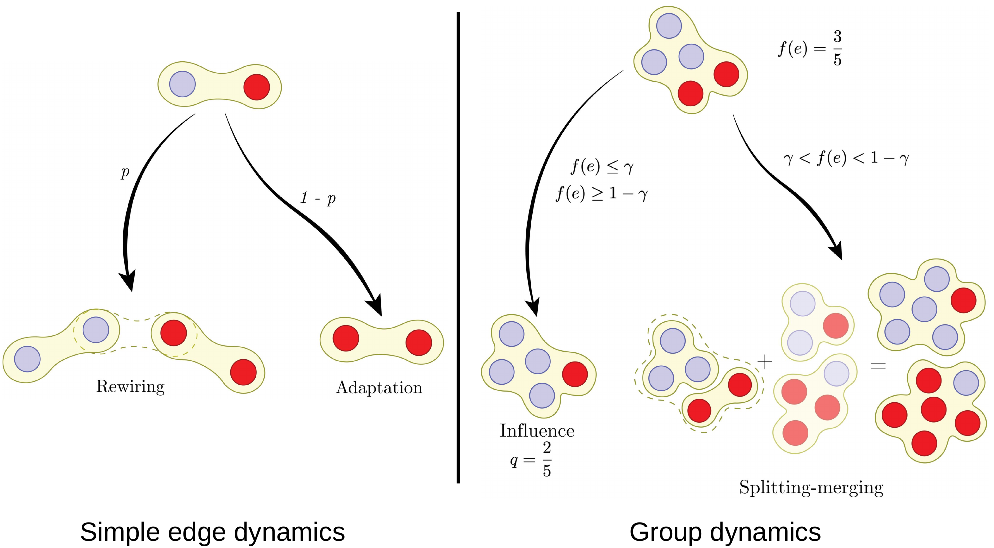# An adaptive voter model on hypergraphs

## May 13, 2022 -

What are the effects of group interactions in opinion formation? Do they help or hamper the emergence of consensus? We study this problem by taking a network perspective and specifically using the adaptive voter model. This is a variation of the voter model where agents can either adopt the opinion of their neighbors or rewire. Here, we extend this model beyond pairwise interactions to include group interactions. For this, we depict the groups as hyperedges. Then, we apply a Heterogeneous Mean Field approximation where agents belong to groups with different sizes, but the distribution of group size is kept constant. In this approximation, we calculate the master equation and quantify the impact of groups. After a detailed analysis, we find that group interactions accelerate the system towards total consensus where one opinion survives. We also prove that the group interactions increase the initial opinion biases, a phenomenon not observed in the classical voter models. Get the article here.